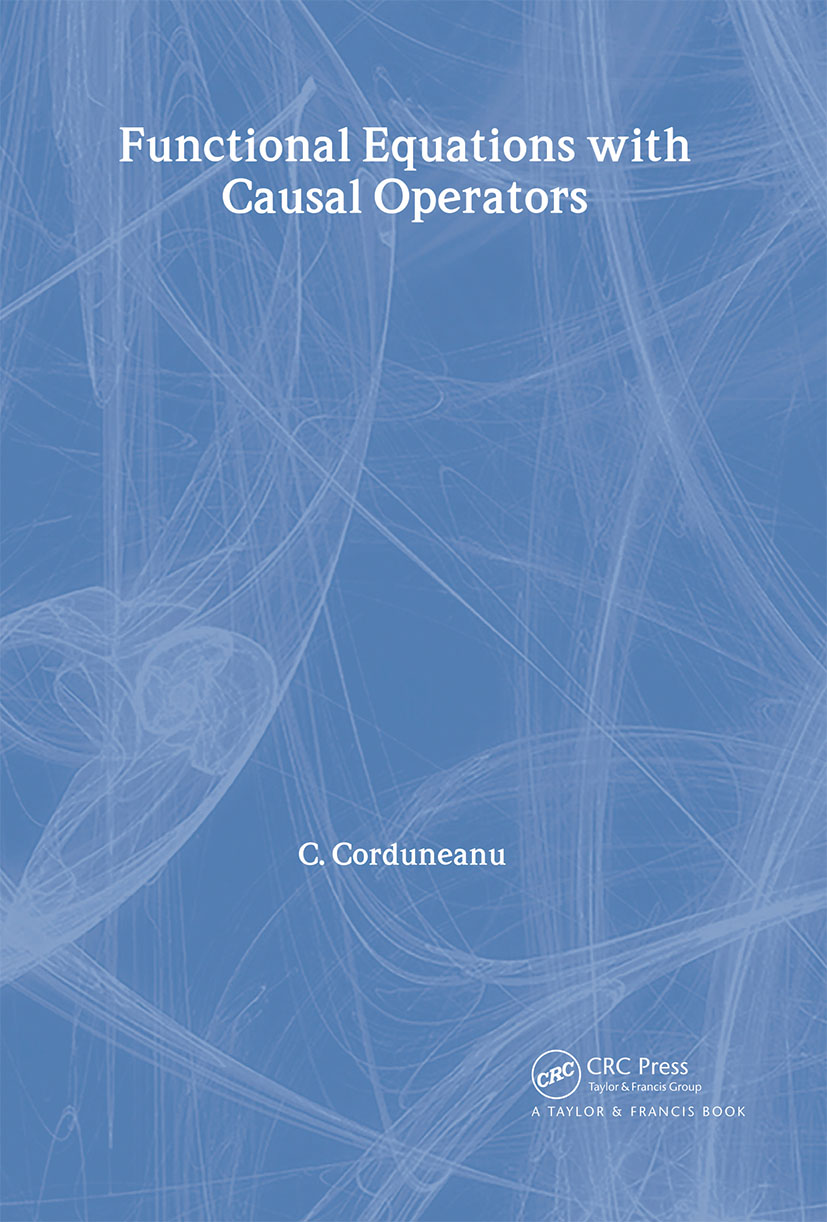# Functional Equations with Causal Operators

## 1st Edition

CRC Press

184 pages

##### Purchasing Options:\$ = USD
Hardback: 9780415271868
pub: 2002-09-05
SAVE ~\$37.00
\$185.00
\$148.00
x
eBook (VitalSource) : 9780429217531
pub: 2002-09-05
from \$28.98

FREE Standard Shipping!

### Description

Functional equations encompass most of the equations used in applied science and engineering: ordinary differential equations, integral equations of the Volterra type, equations with delayed argument, and integro-differential equations of the Volterra type. The basic theory of functional equations includes functional differential equations with causal operators. Functional Equations with Causal Operators explains the connection between equations with causal operators and the classical types of functional equations encountered by mathematicians and engineers. It details the fundamentals of linear equations and stability theory and provides several applications and examples.

Introduction. Auxiliary Concepts. Existence Theory for Functional Equations With Causal Operators. Linear Quasilinear Equations with Causal Operators. Stability Theory. Neutral Functional Equations. Miscellanea (Applications and Generalizations).

### Subject Categories

##### BISAC Subject Codes/Headings:
MAT007000
MATHEMATICS / Differential Equations
MAT037000
MATHEMATICS / Functional Analysis
SCI040000
SCIENCE / Mathematical Physics